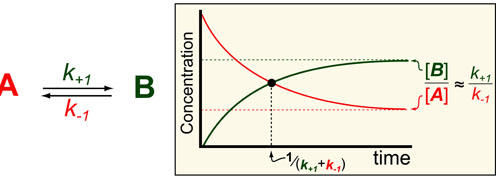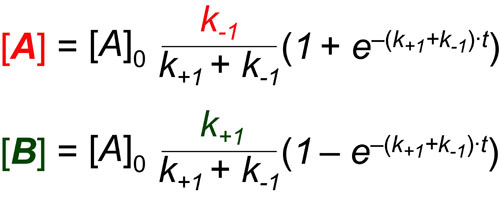# Kinetics #4: Reversible On-Off StatesIn our fourth post in the Understanding Kinetics series we consider the speed at which proteins can turn off (A) or on (B). The dynamics of such processes are important to consider when designing experiments (i.e. How long should I wait to take a measurement?) and understanding Network Motifs in signalling cascades. Luckily we can use exact mathematical models (equations below1) for such processes to define some intuitive rules for the timescales/half-lives and magnitude of these reactions (figure above).

In the scheme above, k+1 and k-1 represent the pseudo-first order rate constants for the conversions of A to B and B to A, respectively. In general, the ratio of A and B at steady-state/equilibrium is equal to the ratio of k+1 to k-1. In addition, the half-life for reaching that steady-state is 1 / (k+1 + k-1). These analysis comes from the mathematical models shown below which are derived in reference 1:### REFERENCES:

1. Banford, C.H.; Tipper, C.F.H. Comprehensive Chemical Kinetics. Elsevier, 1969
2. Segel, I.H. Enzyme Kinetics: Behavior and Analysis of Rapid Equilibrium and Steady-State Enzyme Systems, Wiley-Interscience, 1993
3. Lauffenburger, D.A. Receptors: Models for Binding, Trafficking and Signalling, Oxford University Press 1993.